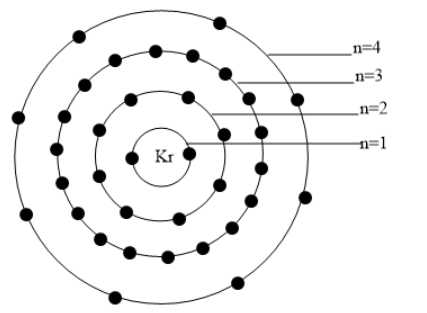# How many valence electrons does Kr have?Verified
179.7k+ views
Hint: The atomic number of Kr is 36.
Kr is a noble gas element and hence they are less reactive or say show no chemical reactions.

So in the question we are asked to calculate the number of valence electrons present in the Kr atom.
Before going into the solution, let’s discuss briefly about what valence electrons are and how it can be determined by knowing the main group number of the elements.
From the lower classes we are learning about the periodic table and the arrangement of the chemical elements according to the increasing order of their atomic number. And we are familiar with the number of groups and periods in the periodic table and also with their group numbers.
Now let’s see what valence electrons mean. The valence electrons are those electrons present in the outermost shell of the atom. These are the electrons which actively take part in the formation of the chemical bonds. So the valence electrons can be said as the electrons present in the valence shell. The valence electrons are either shared or lost to form different types of chemical bonds.
Here we are concerned about the valence electrons in the Kr atom i.e. in Krypton. We know that the atomic number of Kr is 36 and hence they possess 36 electrons in them. And they belong to the group 18 and hence it is a noble gas. Now let’s write the electronic configuration of Kr.
Electronic configuration,of $Kr$=$1{{s}^{2}}2{{s}^{2}}2{{p}^{6}}3{{s}^{2}}3{{p}^{6}}3{{d}^{10}}4{{s}^{2}}4{{p}^{6}}$
Here the valence shell of Kr is having the n (principal quantum number) value 4.
Hence, the outermost shell is having the value n=4.
The valence electrons present in ${{4}^{th}}$ shell is eight.
Therefore, Kr possesses eight valence electrons in their valence shell.
The distribution of electrons in various shells are represented in the figure given below:Note:
We could easily find the valence electrons if we know to which groups the element belongs as the main group number is given according to the valence electrons present in the element.
Example- C belongs to group 14, it is considered and named as the main group, group IV.
Here noble gas belongs to group 18 i.e. main group VIII, hence it has 8 valence electrons.
We should know the increasing order of orbitals to write the electronic configuration of the elements.